# Free Vibration of Conservative Single Degree of Freedom Systems (Part - 1) Civil Engineering (CE) Notes | EduRev

## Civil Engineering (CE) : Free Vibration of Conservative Single Degree of Freedom Systems (Part - 1) Civil Engineering (CE) Notes | EduRev

The document Free Vibration of Conservative Single Degree of Freedom Systems (Part - 1) Civil Engineering (CE) Notes | EduRev is a part of the Civil Engineering (CE) Course Introduction to Dynamics and Vibrations- Notes, Videos, MCQs.
All you need of Civil Engineering (CE) at this link: Civil Engineering (CE)

5.2 Free vibration of conservative, single degree of freedom, linear systems.

• First, we will explain what is meant by the title of this section.
• Recall that a system is conservative if energy is conserved, i.e. potential energy + kinetic energy = constant during motion.
• Free vibration means that no time varying external forces act on the system.
• A system has one degree of freedom if its motion can be completely described by a single scalar variable. We’ll discuss this in a bit more detail later.
• A system is said to be linear if its equation of motion is linear. We will see what this means shortly.

It turns out that all 1DOF, linear conservative systems behave in exactly the same way. By analyzing the motion of one representative system, we can learn about all others.

We will follow standard procedure, and use a spring-mass system as our representative example.

Problem: The figure shows a spring mass system. The spring has stiffness k and unstretched length L0 . The mass is released with velocity v0 from position s0 at time t = 0 . Find s(t) .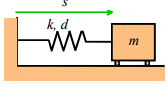There is a standard approach to solving problems like this

(i) Get a differential equation for s using F=ma (or other methods to be discussed)
(ii) Solve the differential equation.

The picture shows a free body diagram for the mass.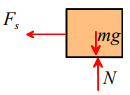Newton’s law of motion states that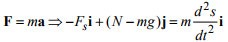The spring force is related to the length of the spring by Fs = k(s -L0). The i component of the equation of motion and this equation then shows that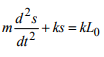This is our equation of motion for s.

Now, we need to solve this equation. We could, of course, use Mathematica to do this – in fact here is the Mathematica solution.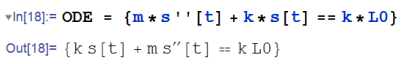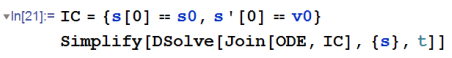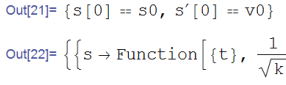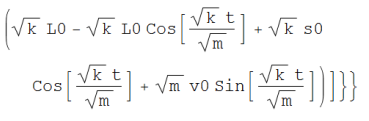This is the correct solution – but Mathematica gives the result in a rather more complicated form than necessary. For nearly all simple vibration problems, it is actually simpler just to write down the solution. We will discuss the general procedure you should follow to do this in the next section.

5.2.1 How to solve equations of motion for vibration problems

Note that all vibrations problems have similar equations of motion. Consequently, we can just solve the equation once, record the solution, and use it to solve any vibration problem we might be interested in. The procedure to solve any vibration problem is:

1. Derive the equation of motion, using Newton’s laws (or sometimes you can use energy methods, as discussed in Section 5.3)
2. Do some algebra to arrange the equation of motion into a standard form
3. Look up the solution to this standard form in a table of solutions to vibration problems.

We have provided a table of standard solutions as a separate document that you can download and print for future reference.

We will illustrate the procedure using many examples

5.2.2 Solution to the equation of motion for an undamped spring-mass system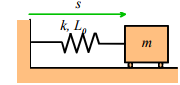We would like to solve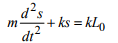with initial conditions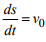from position s0 at time t = 0 .

We therefore consult our list of solutions to differential equations, and observe that it gives the solution to the following equation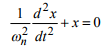This is very similar to our equation, but not identical. To see how to get our equation into this form, note that (i) the standard equation has no coefficient in front of the x; and (ii) its right hand side is zero. We can get our equation to look like this if we divide both sides by k, and subtract d from both sides of the equation. This gives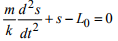Finally, we see that if we define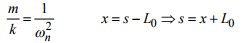then our equation is equivalent to the standard one.

HEALTH WARNING: it is important to note that this substitution only works if L0 is constant, so its time derivative is zero.

The solution for x is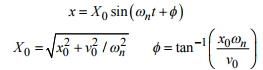Here, x0 and v0 are the initial value of x and dx dt / its time derivative, which must be computed from the initial values of s and its time derivative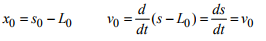When we present the solution, we have a choice of writing down the solution for x, and giving formulas for the various terms in the solution (this is what is usually done):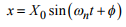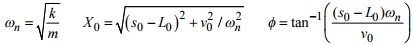Alternatively, we can express all the variables in the standard solution in terms of s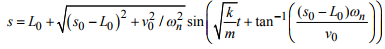But this solution looks very messy (more like the Mathematica solution).

Observe that:

• The mass oscillates harmonically, as discussed in the preceding section;
• The angular frequency of oscillation, ωn, is a characteristic property of the system, and is independent of the initial position or velocity of the mass. This is a very important observation, and we will expand upon it below. The characteristic frequency is known as the natural frequency of the system.
• Increasing the stiffness of the spring increases the natural frequency of the system;
• Increasing the mass reduces the natural frequency of the system

5.2.3 Natural Frequencies and Mode Shapes.

We saw that the spring mass system described in the preceding section likes to vibrate at a characteristic frequency, known as its natural frequency. This turns out to be a property of all stable mechanical systems.

All stable, unforced, mechanical systems vibrate harmonically at certain discrete frequencies, known as natural frequencies of the system.

For the spring—mass system, we found only one natural frequency. More complex systems have several natural frequencies. For example, the system of two masses shown below has two natural frequencies, given by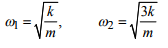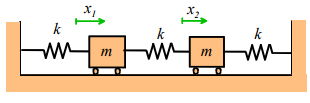A system with three masses would have three natural frequencies, and so on.

In general, a system with more than one natural frequency will not vibrate harmonically.

For example, suppose we start the two mass system vibrating, with initial conditions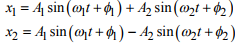with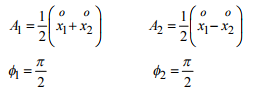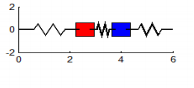In general, the vibration response will look complicated, and is not harmonic. The animation above shows a typical example (if you are using the pdf version of these notes the animation will not work - you can download the matlab code that creates this animation here and run it for yourself)

However, if we choose the special initial conditions: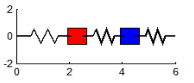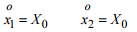then the response is simply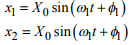i.e., both masses vibrate harmonically, at the first natural frequency, as shown in the animation to the right. (To repeat this in the MATLAB code, edit the file to set A1=0.3 and A2=0)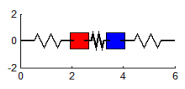Similarly, if we choose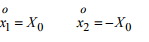then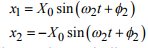i.e., the system vibrates harmonically, at the second natural frequency. (To repeat this in the MATLAB code, edit the file to set A2=0.3 and A1 = 0)

The special initial displacements of a system that cause it to vibrate harmonically are called `mode shapes’ for the system.

If a system has several natural frequencies, there is a corresponding mode of vibration for each natural frequency.

The natural frequencies are arguably the single most important property of any mechanical system. This is because, as we shall see, the natural frequencies coincide (almost) with the system’s resonant frequencies. That is to say, if you apply a time varying force to the system, and choose the frequency of the force to be equal to one of the natural frequencies, you will observe very large amplitude vibrations.

When designing a structure or component, you generally want to control its natural vibration frequencies very carefully. For example, if you wish to stop a system from vibrating, you need to make sure that all its natural frequencies are much greater than the expected frequency of any forces that are likely to act on the structure. If you are designing a vibration isolation platform, you generally want to make its natural frequency much lower than the vibration frequency of the floor that it will stand on. Design codes usually specify allowable ranges for natural frequencies of structures and components.

Once a prototype has been built, it is usual to measure the natural frequencies and mode shapes for a system. This is done by attaching a number of accelerometers to the system, and then hitting it with a hammer (this is usually a regular rubber tipped hammer, which might be instrumented to measure the impulse exerted by the hammer during the impact). By trial and error, one can find a spot to hit the device so as to excite each mode of vibration independent of any other. You can tell when you have found such a spot, because the whole system vibrates harmonically. The natural frequency and mode shape of each vibration mode is then determined from the accelerometer readings.

Impulse hammer tests can even be used on big structures like bridges or buildings – but you need a big hammer. In a recent test on a new cable stayed bridge in France, the bridge was excited by first attaching a

barge to the center span with a high strength cable; then the cable was tightened to raise the barge part way out of the water; then, finally, the cable was released rapidly to set the bridge vibrating.

Offer running on EduRev: Apply code STAYHOME200 to get INR 200 off on our premium plan EduRev Infinity!

## Introduction to Dynamics and Vibrations- Notes, Videos, MCQs

20 videos|53 docs

,

,

,

,

,

,

,

,

,

,

,

,

,

,

,

,

,

,

,

,

,

;